### Home > PC > Chapter 4 > Lesson 4.1.2 > Problem4-28

4-28.
1. The amplitude of a periodic function is defined as half the distance between the highest point (or max) and the lowest point (or min). It can also be thought of as the distance from the middle of the graph to the top. Consider the “sawtooth” graph at right. Homework Help ✎

1. What is the amplitude?

2. What is the period?

3. Find the domain and range.

4. What are the roots of the function? Express the roots as an equation.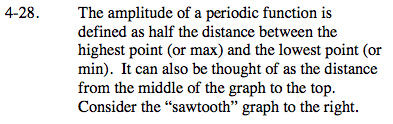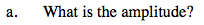2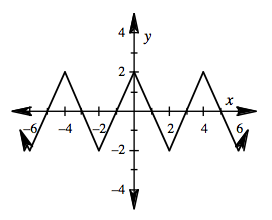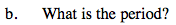4

The period is the horizontal distance to complete 1 cycle.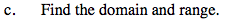All Real numbers.

[−2, 2]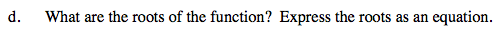... –5, –3, –1, 1, 3, 5, ...